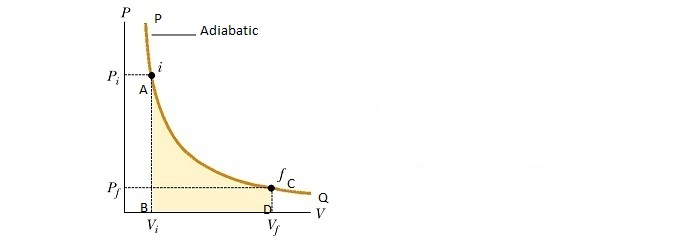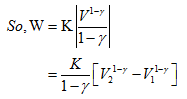Click to Chat

1800-1023-196

+91-120-4616500

CART 0

• 0

MY CART (5)

Use Coupon: CART20 and get 20% off on all online Study Material

ITEM
DETAILS
MRP
DISCOUNT
FINAL PRICE
Total Price: Rs.

There are no items in this cart.
Continue Shopping• Complete Physics Course - Class 11
• OFFERED PRICE: Rs. 2,968
• View Details

Work Done During Adiabatic Expansion

Table of Content

Graphical Method

Analytical Method

Solved Problems

Related Resources

Consider one gram molecule of a perfect gas, enclosed in a perfectly non-conducting cylinder provided with a non-conducting piston. If the piston is now moved suddenly outwards, the gas expands, and does some work. Since no heat supplied from outside, adiabatic expansion has occurred. Therefore, the energy required for the expansion of the gas is taken from the gas itself so that its temperature falls.Let the piston of area of cross section ‘A’ move through a small distance dx, so that the gas expands by a small amount dV. For a small expansion of the gas, pressure remains, practically constant, say P. Then

the force acting on the piston,

F = PA

and small work done by the gas,

dW = F dx

= PA dx

= p dV        (Since, dW = P dV)(a) Graphical Method

This case is different from the case of isothermal change in two respects.

(i) The container is made up an insulating material.

(ii) The curve is much sharper.

The adiabatic curve PQ for the process is shown in below figure.
The net work done to expand the gas from a volume V1 to volume V2 will be,
W = Area ABDC
Thus, area occupied below the curve in between AB and CD gives the required value of work done.

(b) Analytical Method

Analytically, net work W can be calculated by integrating dW between the limits V1 and V2.

PVγ = constant = K (say)

Or, P = K / Vγ

Equation (1) gives,Now P1V1γ  = P2V2γ =K

So, W = [1/1-γ] [P2  V2γ  V2-γ1 - P1 V1γ V11-γ]

Or, W = [1/1-γ] [P2V2 – P1V1]       ------- (2)

If T1 and T2 are the initial and final temperatures of the gas,

P1V1 = RT1 and P2V2 = RT2

Substituting in equation (2) we get,

W = [R/1-γ] [T2 - T1]

Or, W = [R/γ-1] [T1 - T2]                 --------(3)

Therefore, heat required to do the work during expansion is,

H = W/J

= [R / J(γ-1)] [T1 - T2]

As R is gas constant and γ is constant for a given gas, therefore, we find, from equation (3), that work done during an adiabatic expansion is proportional to the fall in temperature. On the other hand, if the gas is compressed, the work is done on the gas and is negative. The work done for adiabatic compression is given by,

W =  – [R/γ-1] [T1 - T2]

Or, W = [R/γ-1] [T2 - T1]          ------(4)

and

H =  [R/γ-1] [T2 - T1]

Solved Problems

Problem 1:-

The gas in a cloud chamber at a temperature of 292 K undergoes a rapid expansion. Assuming the process is adiabatic, calculate the final temperature if γ = 1.40 and the volume expansion ratio is 1.28.

Concept:-

The relation between temperature T and volume V from the adiabatic gas equation is,

T V γ-1 = constant

Where T is the temperature and V is the volume of the gas.

Since the gas expand is expanding from its initial volume Vi and its initial temperature Ti to its final volume Vf and its final temperature Tf, therefore the equation (1) will be,

Ti Vi γ-1 = Tf Vf γ-1

So,

Tf = Ti (Vi/Vf) γ-1

Solution:-

To find out the final temperature of the gas Tf, substitute 292 K for Ti, 1.28 for volume expansion ratio Vf/Vi and 1.40 for γ in the equation Tf = Ti (Vi/Vf) γ-1,

Tf = Ti (Vi/Vf) γ-1

= 292 K (1/1.28)1.40-1

= 265 K

From the above observation we conclude that, after expansion the final temperature of the gas will be 265 K.

Problem 2:-

In a motorcycle engine, after combustion occurs in the top of the cylinder, the piston is forced down as the mixture of gaseous products undergoes an adiabatic expansion. Find the average power involved in this expansion when the engine is running at 4000 rpm, assuming that the gauge pressure immediately after combustion is 15.0 atm, the initail volume is 50.0 cm3, and the volume of the mixture at the bottom of the stroke is 250 cm3. Assume that the gases are diatomic and that the time involved in the expansion is one-half that of the total cycle.

Concept:-

In an adiabatic process, the final pressure pf is defined as,

pf = piViγ/Vfγ

= pi [Vi/Vf]γ

The work done W for adiabatic process is defined as,

W = [pfVf - piVi]/(γ-1)

Here, initial pressure is pi, initial volume is Vi and final volume is Vf and adiabatic constant is γ.

Solution:-

First we have to find out final pressure pf. To obtain pf, substitute 16.0 atm for pi, 50.0 cm3 for Vi, 250 cm3 for Vf and 1.40 for γ in the equation pf = pi [Vi/Vf]γ,

pf = pi [Vi/Vf]γ

=(16.0 atm) [50/250]1.40

= 1.68 atm

To obtain the work done W by the gas during the expansion, substitute 16.0 atm for pi, 50.0 cm3 for Vi,1.68 atm for pf, 250 cm3 for Vf and 1.40 for γ in the equation W = [pfVf - piVi]/(γ-1), we get,

W = [pfVf - piVi]/(γ-1)

= (16.0 atm) (50.0 cm3) – (1.68 atm) (250 cm3)/(1.40-1)

= [(16.0 atm×(1.01×105 Pa/1 atm)) (50.0 cm3×(10-6 m3/1 cm3))] –[ (1.68 atm×(1.01×105 Pa/1 atm)) (250 cm3×(10-6 m3/1 cm3))]/(1.40-1)

=96.0 Pa.m3

= (96.0 Pa.m3) (1 N/m2/ 1 Pa)

= (96.0 N.m) (1 J/ 1 N.m)

= 96.0 J

The above process happens 4000 times per minute, but the actual time to complete the process is half of the cycle, or 1/8000 of a minute.

Thus the average power P involved in this expansion when the engine is running at 4000 rpm will be,

P = (96.0 J) (8000)/(60 s)

= 12.8×103 J/s

= (12.8×103 J/s) (1 W/1 J/s)

= 12.8×103 W

From the above observation we conclude that, the average power P involved in this expansion when the engine is running at 4000 rpm would be 12.8×103 W.

Problem 3:-

In a steam locomotive, steam at boiler pressure of 16.0 atm enters the cylinder, is expanded adiabatically to 5.60 times its original volume, and then exhausted to the atmosphere. Calculate (a) the steam pressure after expansion and (b) the greatest possible efficiency of the engine.

Concept:-

pV γ = constant

Here p is the pressure of the gas and V is the volume of the gas.

If the gas expands from its initial state (p1,V1) to its final state (p2,V2), then,

p1 V1  γ = p2 V2  γ

So the final pressure p2 of the gas will be,

p2 = p1 (V1/ V2) γ

From adiabatic gas equation we know that,

TV γ-1 = constant

If the gas expands from its initial state (T1,V1) to its final state (T2,V2), then,

T1 V1 γ-1 = T2 V2 γ-1

So the final temperature T2 will be,

T2 = T1 (V1/V2) γ-1

So, T2/T1 = (V1/V2) γ-1

For ideal polyatomic gas the value of γ is equal to 1.33.

The efficiency e of an engine is defined as,

e = 1-(T2/T1)

Solution:-

(a) Let the initial volume of the steam is V1.

After expansion its original volume is 5.60 times of the initial volume. Therefore the final volume V2 of the steam will be,

V2 = 5.60 V1

To find out the final pressure p2 of the steam after expansion, substitute 16.0 atm for p1, 5.60 V1 for V2 and 1.33 for γ in the equation p2 = p1 (V1/ V2)  γ,

p2 = p1 (V1/ V2) γ

= (16.0 atm) (V1/5.60 V1) 1.33

= (16.0 atm) (1/5.60) 1.33

= 1.62 atm

From the above observation we conclude that, the final pressure p2 of the steam after expansion will be 1.62 atm.

(b) To obtain the greatest possible efficiency e of the engine, first we calculate the final temperature ratio T2/T1 of the steam.

To find out the final temperature ratio T2/T1, substitute 5.60 V1 for V2 and 1.33 for γ in the equation T2/T1 = (V1/V2) γ-1,

T2/T1 = (V1/V2) γ-1

= (V1/5.60 V1) 1.33-1

= (1/5.60) 0.33

= 0.567

To obtain the greatest possible efficiency e of the engine, substitute 0.567 for T2/T1 in the equation e = 1-(T2/T1),

e = 1-(T2/T1)

= 1-0.567

= 0.433

From the above observation we conclude that, the greatest possible efficiency e of the engine would be 0.433.

Related Resources

Look into the Sample Papers of Previous Years to get a hint of the kinds of questions asked in the exam.

To read more, Buy study materials of Thermodynamics comprising study notes, revision notes, video lectures, previous year solved questions etc. Also browse for more study materials on Chemistry here.### Course Features

• 101 Video Lectures
• Revision Notes
• Previous Year Papers
• Mind Map
• Study Planner
• NCERT Solutions
• Discussion Forum
• Test paper with Video Solution